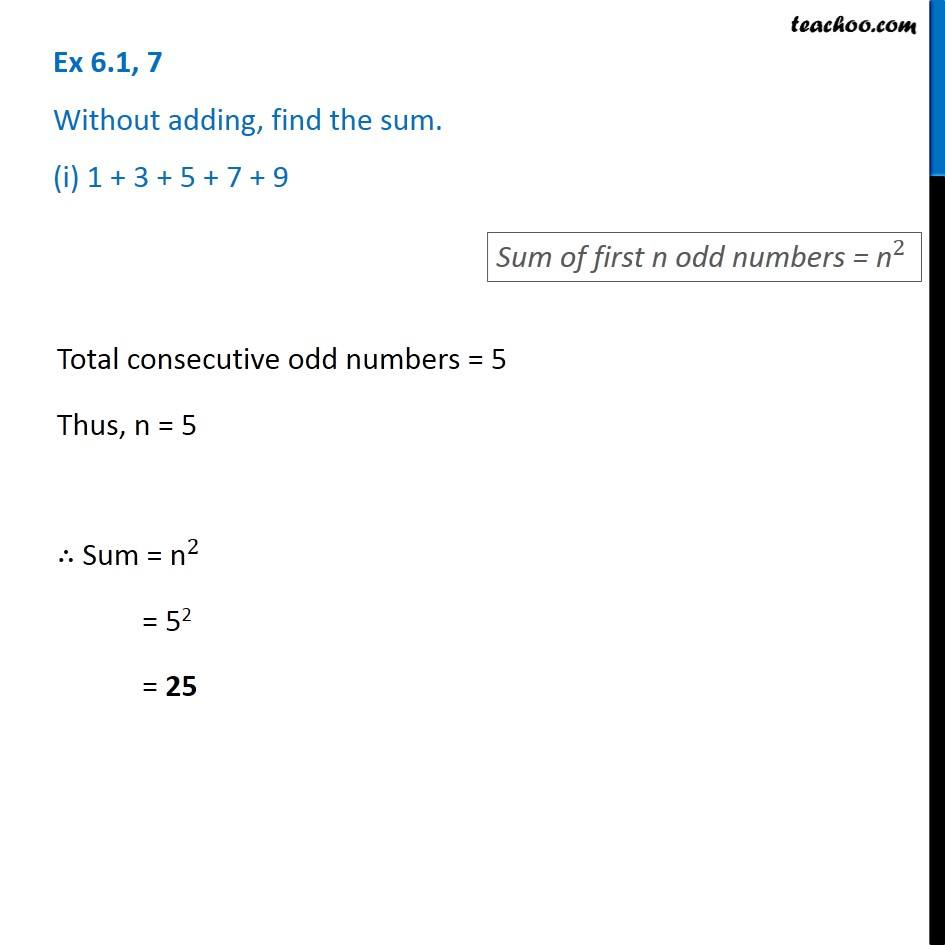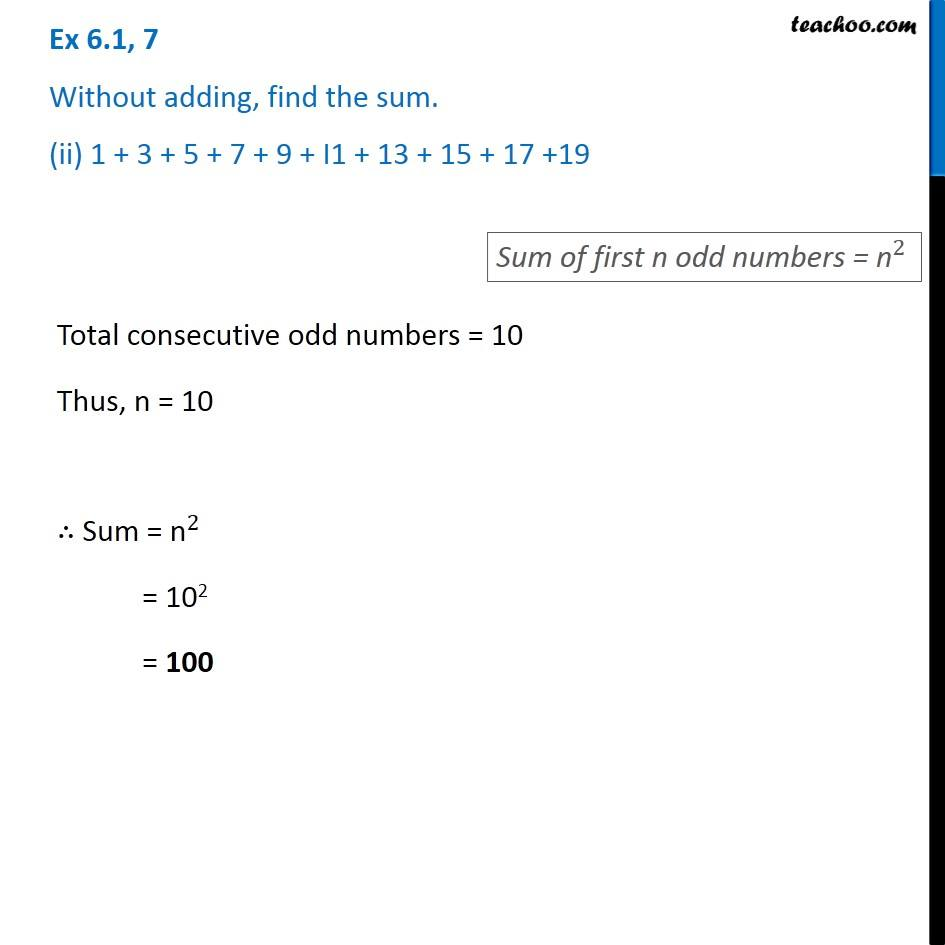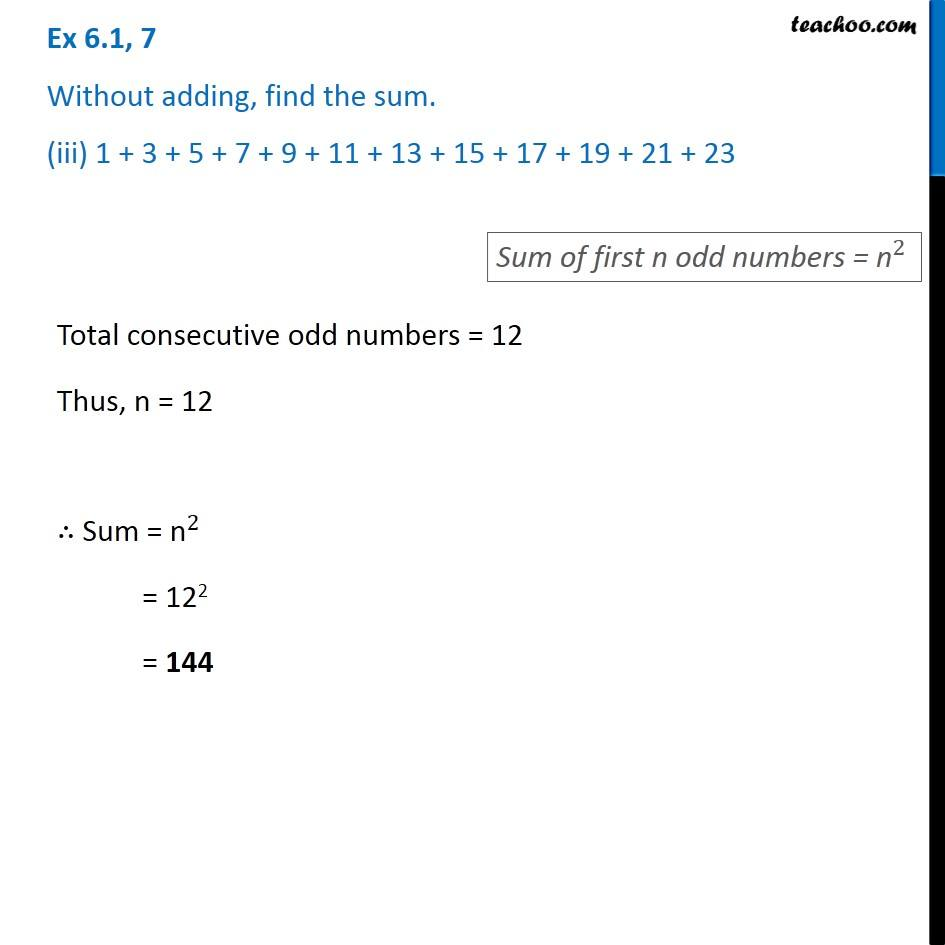1. Chapter 6 Class 8 Squares and Square Roots
2. Serial order wise
3. Ex 6.1

Transcript

Ex 6.1, 7 Without adding, find the sum. (i) 1 + 3 + 5 + 7 + 9 Total consecutive odd numbers = 5 Thus, n = 5 ∴ Sum = "n" ^2 = 52 = 25 Ex 6.1, 7 Without adding, find the sum. (ii) 1 + 3 + 5 + 7 + 9 + I1 + 13 + 15 + 17 +19 Total consecutive odd numbers = 10 Thus, n = 10 ∴ Sum = "n" ^2 = 102 = 100 Ex 6.1, 7 Without adding, find the sum. (iii) 1 + 3 + 5 + 7 + 9 + 11 + 13 + 15 + 17 + 19 + 21 + 23 Total consecutive odd numbers = 12 Thus, n = 12 ∴ Sum = "n" ^2 = 122 = 144

Ex 6.1